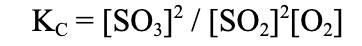• Call Now

1800-102-2727•

# Homogeneous Equilibrium

Equilibrium is a chemical reaction state in which the backward and forward reaction rates are equal. Furthermore, there are two forms of equilibrium: heterogeneous equilibrium and homogeneous equilibrium. In one phase, a homogeneous mixture (products and reactants in a single solution) is defined as a homogeneous equilibrium. Keep in mind that the reactants are on the left side of the equation and the products are on the right side of the equation. As a result, the reaction that occurs between the solutes is part of a single homogeneous equilibrium. A heterogeneous equilibrium, on the contrary, is a reaction system in which the reactants and the products are distributed across two or more phases.

## Chemical Equilibrium That Is Homogeneous

It's vital to notice that the two different equilibriums,and the calculations associated with them, are treated differently. A chemical equation concerning homogeneous equilibrium is presented below to help you understand it better.

C2H2 (aq) + 2Br2 (aq) ⇌ C2H2Br2 (aq)

In order to understand the distinction between heterogeneous and homogeneous equilibrium, a heterogeneous equilibrium example is also given. The following is an example:

H2O (s) ⇌ H2O (l)

## Example of Homogeneous Equilibrium

A homogeneous equilibrium can also be classified into two types. The number of molecules in the product is the same as the number of molecules in the reactants of that particular equation in the first category.

For example: N2 (g) + O2 (g) = 2NO (g)

We can see in the example above that there are two molecules of reactants (one of each) and two molecules of product on the right side. The opposite situations are encountered in the second category of a homogeneous equilibrium equation. The product's number of molecules is not the same as or equal to the reactant's number of molecules.
For example:

2SO2 (g) + O2 (g) ⇌ 2SO3 (g)

We can see from the previous example that the reaction contains just three molecules of reactant and only two molecules of product.

## Determine the Equilibrium Constant

For a given reaction, you can compute or solve the equilibrium constant. Let's use the hypothetical products and reactants W, X, Y, and Z to demonstrate this. w, x, y, and z represent their coefficient, which is the number in front of the molecule or component. The following is the equation for these compounds and their coefficient:

wW + xX ⇌ yY +zZ

The products of the equation go in the numerator, with the coefficient as their exponent, to determine the equilibrium constant of this and similar equations. The reactants, on the other hand, are placed in the numerator, with their coefficients serving as exponents. The written expression will resemble the following:

Any equation's equilibrium constant can be calculated using this formula. The following is another example of a chemical equation that can be used to explain:
Equation

2SO2 (g) + O2 (g) ⇌ 2SO3 (g)

## Equilibrium ConstantFurthermore, partial pressure is taken into account while determining the equilibrium constant for gases. The ideal gas equation is used as the first stage in this process. The ideal gas equation's formula is as follows:

PV = nRT; where
P = pressure,
V = volume
N = number of moles of components
R = the universal gas constant
T = temperature

The above equation can also be written as:
P = (n/v)RT
where,
n/v = c (concentration of the system)
P = cRT
As a result, the equilibrium constant can be represented in the formula using the system's concentration. The steps below can be used to derive this relationship.

KP = KC(RT)Δn
where
Δn = the number of moles of gas,
KP - is defined as the equilibrium constant determined from a reaction equation's partial pressure.

## Difference Between KC and KP, there's a lot to choose from.

The most significant distinction between the two equilibrium constants is that they are employed for different concentrations. During a reaction, KP stands for the equilibrium constant at partial pressure. These values can be calculated from the products and reactants, and the equations and the exact values of those formulae. There is also a relationship between the two equilibrium constants, which is presented below:
KP = KC(RT)ΔnTalk to our expert
Resend OTP Timer =
By submitting up, I agree to receive all the Whatsapp communication on my registered number and Aakash terms and conditions and privacy policy